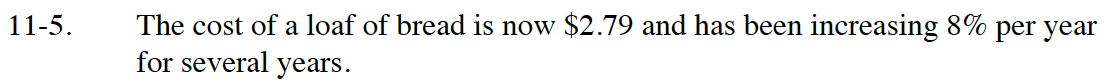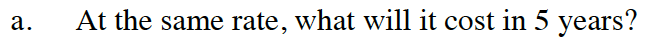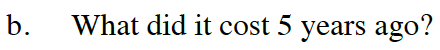Home > CC4 > Chapter 11 > Lesson 11.1.1 > Problem11-5

11-5.
1. The cost of a loaf of bread is now $2.79 and has been increasing 8% per year for several years. Homework Help ✎ 1. At the same rate, what will it cost in 5 years? 2. What did it cost 5 years ago?What is the multiplier? Use the multiplier to calculate your answer.$4.10Think of this as a geometric sequence.
Going forwards (in time) you multiply.
How would you go backwards (in time)?